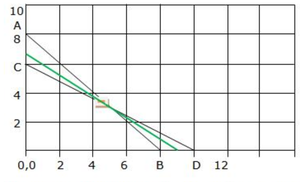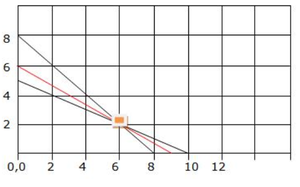Open in App
Not now

# Class 12 RD Sharma Solutions- Chapter 30 Linear Programming – Exercise 30.1 | Set 2

• Last Updated : 15 Feb, 2023

### Question 11. Two tailors A and B earn Rs 150 and Rs 200 per day respectively. A can stitch 6 shirts and 4 pants per day while B can stitch shirts and 4 pants per day. Form a linear programming problem to minimize the labour cost to produce at least 60 shirts and 32 pants.

The above LPP can be represented in the table above.

To minimize labour cost means to assume minimize the earnings, i.e, Min Z = 150x + 200y such that the constraints

x>=0; y >= 0  (at least 1 shirt and pant is required)

6x + 10y >= 60  (require at least 60 shirts)

4x + 4y >= 32  (require at least 32 pants)

Solving the above inequalities as equations we get, x = 5 and y = 3

Other corner points obtained are (0, 6) & (10, 0), (0, 8) & (8, 0)The feasible region is the open unbounded region A-E-D.

Point E(5, 3) may not be the minimal value. So, plot 150x + 200y < 1350 to see if there is a common region with A-E-D.

The green line has no common point, therefore

Stitching 5 shirts and 3 pants minimizes labour cost to Rs. 1350.

### Given information can be tabulated as below:

The above LPP can be represented in the table above.

The flight cost is to be minimized, i.e, Min Z = x +1.5y such that the constraints

x >= 2  (at least 2 planes of model 314 must be used)

y >= 0  (at least 1 planes of model 535 must be used)

20x + 20y >= 160  (require at least 160F class seats)

30x + 60y >= 300  (require at least 300T class seats)

Solving the above inequalities as equations we get,

When x = 0, y = 8 and when y = 0, x = 8

When x = 0, y = 5 and when y = 0, x = 10

We get an unbounded region 8-E-10 as a feasible solution. Plotting the corner points and evaluating we have,

Since we obtained an unbounded region as the feasible solution a plot of Z ( x + 1.5y < 9) is plotted.

since there are no common points , point E is the point that gives the minimum value.

Using 6 planes of model 314 & 2 of model 535 gives minimum cost of 9 lakh rupees.### Question 13. Amit’s mathematics teacher has given him three very long list of problems with the instruction to submit not more than 100 of them (correctly solved) for credit. The problem in the first set are worth 5 points each, those in the second set are worth 4 points each, and those in the third set are worth 6 points each. Amt knows from experience that he requires on the average 3 minutes to solve a 5 points problem, 2 minutes to solve a 4 points problem, and 4 minutes to solve a 6 point problem. Because he has other subjects to worry about, he cannot afford to devote more than 3.5 hours altogether to his mathematics assignment. Moreover, the first two sets of problems involve numerical calculations and he knows that he cannot stand more than 2.5 hours work on this type of problem. Under these circumstances, how many problems in each of these categories shall he do in order to get maximum possible credit for his efforts? Formulate this as a LPP.

Given information can be tabulated as below

Let there be x, y, z questions from set 1, 2 and 3 respectively.

Given, each question from set 1, 2 and 3 earn 5,4 and 6 points respectively. So x questions of set 1, y questions of set 2 and z questions of set 3 earn 5x, 4y and 6z points.

Let total point credit be U

So, U = 5x + 4y + 6z

Given, each questions of set 1, 2 and 3 require 3, 2 and 4 minutes respectively. So, x questions of set 1, y questions of set 2 and z questions of set 3 require 3x, 2y and 4z minutes respectively but given that total time to devote in all three sets is 3.5 hours = 210 minutes and the first two sets is 2.5 hours = 150 minutes.

So,

3x + 2y + 4z <= 210 (First constraint)

3x + 2y < =150  (Second constraint)

Given, total number of questions cannot exceed 100.

So, x + y + z <= 100   (Third constraint)

Hence, mathematical formulation of LPP is

Find x and y which maximize U = 5x + 4y + 6z

Subject to constraint,

3x + 2y + 4z <= 210

3x + 2y < =150

x + y + z <= 100

x, y, z >= 0 (Since number of questions to solve from each set cannot be less than zero)

### Question 14. A farmer has a 100 acre farm. He can sell the tomatoes, lettuce or radishes he can raise. The price he can obtain is Re 1 per kilogram for tomatoes, Rs 0.75 a head for lettuce and Rs 2 per kilogram for radishes. The average yield per acre is 2000 kgs for radishes , 3000 heads of lettuce and 1000 kilograms of radishes. Fertilizer is available at Rs 0.05 per kg and the amount required per acre is 100 kgs each for tomatoes and lettuce and 50 kilograms for radishes. Labour required for sowing, cultivating and harvesting per acre is 5 man days for tomatoes and radishes and 6 man days for lettuce. A total of 400 man days of labour are available at Rs 20 per man day. Formulate this problem as a LPP to maximize the farmer’s total profit.

Given information can be tabulated as below:

Average 2000 kg/per acre

Total land = 100 Acre

Cost of fertilizers = Rs 0.50 per kg

A total of 400 days of cultivation labour with Rs 20 per day

Let required quantity of field for tomatoes, lettuce and radishes be x, y and z acre respectively.

Given, costs of cultivation and harvesting of tomatoes, lettuce and radishes are 5 * 20 = Rs 100, 6 * 20 = Rs 120, 5 * 20 = Rs 100 respectively per acre. Cost of fertilizers for tomatoes, lettuce and radishes 100 * 0.05 = Rs 50, 100 * 0.50 = Rs 50 ans 50 * 0.50 = Rs 25 respectively per acre.

So, total costs of production of tomatoes, lettuce and radishes are Rs 100 + 50 = Rs 150x, Rs 120 + 50 = Rs 170y and Rs 100 + 25 = Rs 125z respectively. Total selling price of tomatoes, lettuce according to yield are 2000 * 1 = Rs 2000x, 3000 * 0.75 = Rs 2250y and 1000 * 2 = Rs 2000z respectively.

Let U be the total profit,

So,

U = (2000x – 150x) + (2250y – 170y) + (2000z – 125z)

U = 1850x + 2080y + 1875z

Given, farmer has 100 acre from

So, x + y + z <= 100 (First constraint)

Number of cultivation and harvesting days are 400

So, 5x + 6y + 5z <= 400  (Second constraint)

Hence, mathematical formulation of LPP is

Find x, y, z which maximize U = 11850x + 2080y + 1875z

Subject to constraint,

x + y + z <= 100

5x + 6y + 5z <= 400

x, y, z >=0  (Since cultivation cannot be less than zero)

### The problem is to determine the number of units to produce each product so as to maximize total contribution to profit. Formulate this as a LPP.

Given information can be tabulated as below:

Let the required number of product A and B be x and y units respectively.

Given, labour cost and raw material cost of one unit of product A is Rs 16 and Rs 4, so total cost of product A is Rs 16 + Rs 4 = Rs 20

And given selling price of 1 unit of product A is Rs 25

So, profit on one unit of product A = Rs 25 – Rs 20 = Rs 5

Again,  labour cost and raw material cost of one unit of product A is Rs 20 and Rs 4, so total cost of product A is Rs 20 + Rs 4 = Rs 24

And given selling price of 1 unit of product B is Rs 30

So, profit on one unit of product B = Rs 30 – Rs 24 = Rs 6

Hence, profits on x unit of product A and y units of product B are Rs 5x and Rs 6y respectively.

Let Z be the total profit, so Z = 5x + 6y

Given, production of one unit of product A and B need to process for 3 and 4 hours respectively in department 1, so production of x units of product A and y units of product B need to process for 3x and 4y hours respectively in department 1. But total capacity of Department 1 is 130 hours,

So, 3x + 2y <= 130  (First Constraint)

Given, production of one unit of product A and B need to process for 4 and 6 hours respectively in department 2, so production of x units of product A and y units of product B need to process for 4x and 6y hours respectively in department 2. But total capacity of Department 2 is 260 hours,

So, 4x + 6y <= 260  (Second Constraint)

Hence, mathematical formulation of LPP is

Find x, y, z which maximize Z = 5x + 6y

Subject to constraint,

3x + 2y <= 130

4x + 6y <= 260

x, y >= 0 (Since production cannot be less than zero)

My Personal Notes arrow_drop_up
Related Articles